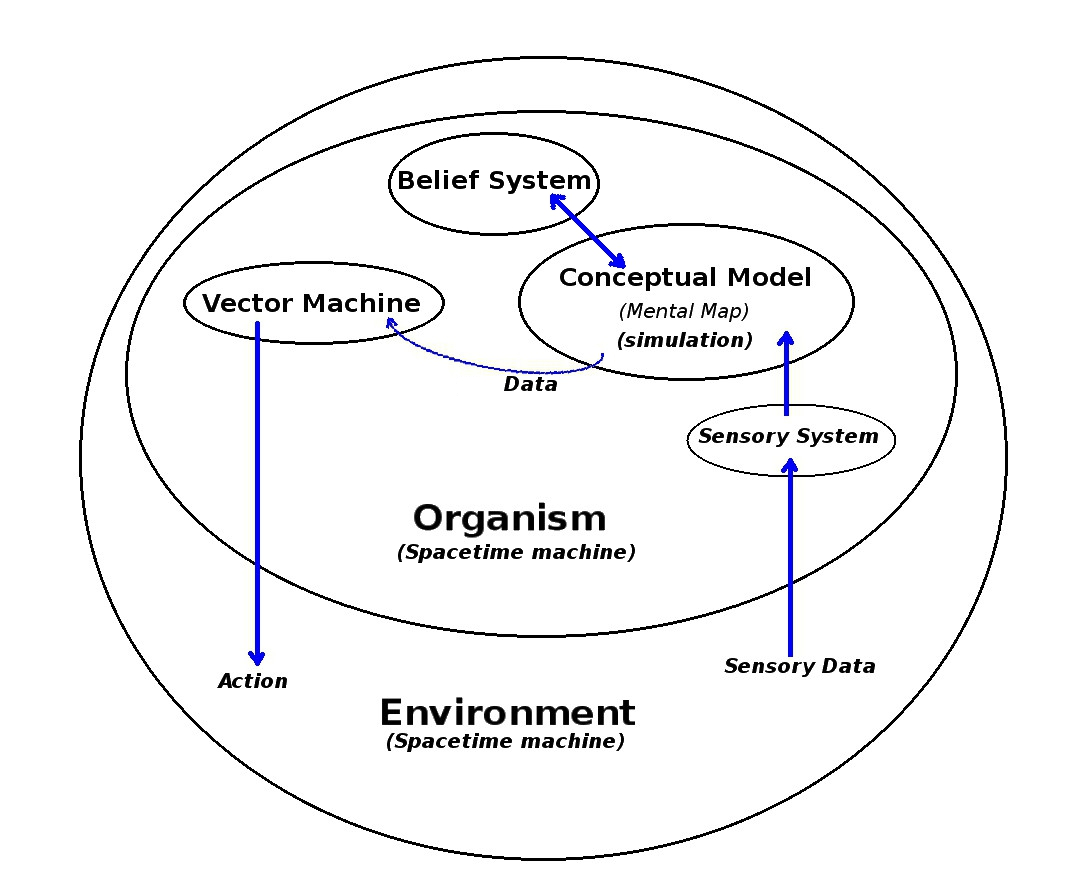# Mental Maps and Vectored Forces:

## Terminolgy:

A Machine

(in this context) is a system that "specifically does something"
-it can be either a natural system or a man made one.

A System

(in this context) is a coordinated collection of components -operating together to form a single unit

A Spacetime Machine

(in this context) is a system that moves through time and space modifying the space in which it moves
(see The Philosophy of Water)

A Vector

(in this context) is a directional line of force
(i.e. a certain amount of pressure (force) is assumed to be exerted in a certain direction)

A Line of Least Resistance

(in this context) is a line of  action (vector) that (in a specified context) will create the least resistance when carried out.

A Vector Machine

(in this context) is a system that calculates vectors -based on a set of values provided by some source
These "values" can be completely subjective -and can be in any number of domains -i.e. economic, political, moral, philosophical or psychological, etc

A Belief System

(in this context) is a set of beliefs (practical, theoretical, moral, religious, etc.) that provide the value system used to calculate the line of least resistance.
The belief system is both a consequence of the Mental Map
-and the psychological (or practical) driving force behind its creation.

A Mental Map

(in this context) is a mental image (model) of the environment being acted on

-It provides the data for calculating the vector which specifies which action (the line of least resistance) is to be taken (under the current circumstances in the specified environment given the perceived set of choices implied by the model).

### If a Vector Machine calculates the Line of Least Resistance   -on what basis is the calculation made?

The calculation is made on the basis of the subjective values embedded in the Mental map## Integrating (Cognitive) Mental maps with (Emotional) Vector Forces

-------------------------------------------------

Think Tank
The Philosophy of Water
Does it Matter if Things go Wrong?

-------------------------------------------------

Trevor Batten
<trevor at tebatt dot net>
Baclayon 2019
home